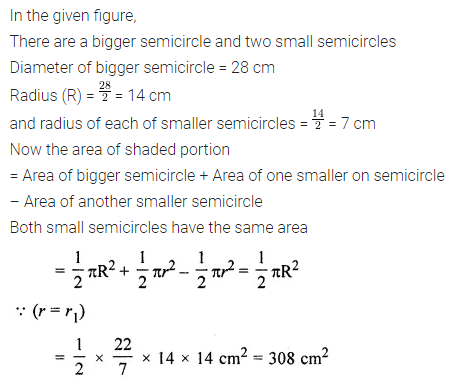# ML Aggarwal Class 7 Solutions for ICSE Maths Chapter 16 Perimeter and Area Check Your Progress

## ML Aggarwal Class 7 Solutions for ICSE Maths Chapter 16 Perimeter and Area Check Your Progress

Question 1.
A 3 m wide path runs outside and around a rectangular park of length 125 m and breadth 65 m. Find the area of the path.
Solution:Question 2.
In the given figure, all adjacent line segments are at right angles. Find:
(i) the area of the shaded region
(ii) the area of the unshaded region.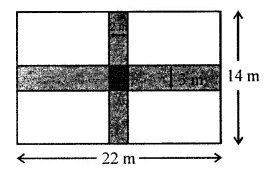Solution:Question 3.
Find the area of a triangle whose:
(i) base = 2 m, height = 1.5 m
(ii) base = 3.4 m and height = 90 cm
Solution:Question 4.
In the given figure, PQRS is a parallelogram. QM is the height from Q to SR and QN is the height from Q to PS. If SR = 12 cm, PS = 8 cm and QM = 7.6 cm, find:
(i) the area of the parallelogram PQRS
(ii) the length of QN.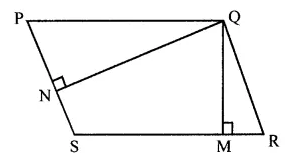Solution:Question 5.
From the given figure, find
(i) the area of ΔABC
(ii) length of BC
(iii) the length of altitude from A to BC.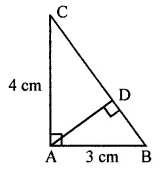Solution:Question 6.
In the given figure, the area of the right-angled triangle is 54 cm2. If one of its legs is 12 cm long, find its perimeter.Solution:Question 7.
If the area of a circle is 78.5 cm2, find its circumference. (Take π = 3.14)
Solution:Question 8.
Find the circumference of the circle whose area is 16 times the area of the circle with diameter 7 cm.
Solution: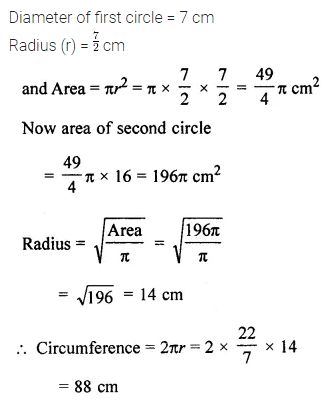Question 9.
From square cardboard, a circle of the biggest area was cut out. If the area of the circle is 154 cm2, calculate the original area of the cardboard.
Solution: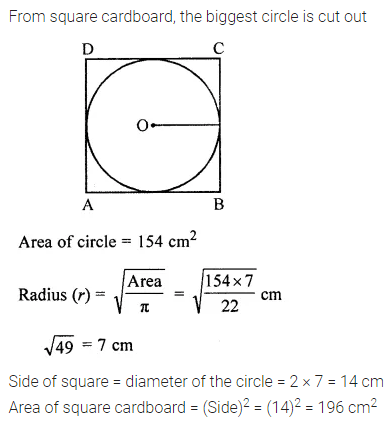Question 10.
A road 3.5 m wide surrounds a circular park whose circumference is 88 m. Find the cost of paving the road at the rate of ₹ 60 per square meter.
Solution: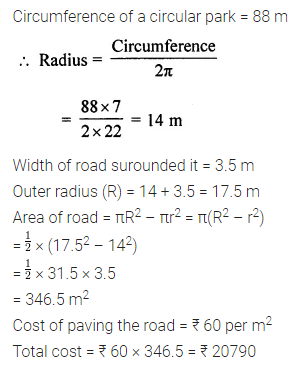Question 11.
In the given figure, ABCD is a square of side 14 cm. Find the area of the shaded region.
Take π = $$\frac { 22 }{ 7 }$$Solution: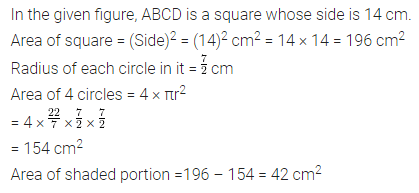Question 12.
The boundary of a shaded region in the given figure consists of three semicircles, the smaller being equal. If the diameter of the larger one is 28 cm, find
(i) the length of the boundary
(ii) the area of the shaded region.Solution: